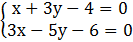### Sample Problem

Solve using the substitution method:x=

y=

#### Solutionx=4-3y (from the first equation) – now substitute x=4-3y into the second equation

3(4-3y)-5y-6=0

12-9y-5y-6=0

6=14y

6/14=3/7=y

By back substituting y=3/7 into either equation, we can solve for x.

x+3y-4=0

x=4-3y=4-3(3/7)=19/7

x=19/7 ; y=3/7